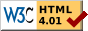## Dokumentation zu: strftime(E)``` SYNOPSIS string strftime() string strftime(string fmt) string strftime(int clock) string strftime(string fmt, int clock) string strftime(string fmt, int clock, int localized) DESCRIPTION The function strftime() formats the given time in as a formatted string, similar to ctime(). Unlike ctime(), strftime() accepts a format string with placeholders for the different elements. The format string consists of zero or more conversion specifiers (see below) and ordinary characters. Ordinary charaecters are just copied to the result. A conversion specifier starts with a percent sign ('%'). If no format string is given, a default of "%c" will be used. The argument is the number of seconds since the epoch (00:00:00 UTC, January 1, 1970), as returned by time/mktime(). If is not given, the current result of time() will be used. The argument specifies, whether the result will be in english (the classic "C" locale) or in the language configured on the host computer (e.g. german). The language has to be configured with the environment variables LC_TIME or LC_ALL (please ask your admins). If is not given, the default is 1. 0: use classic "C" locale (english) 1: use current locale on the host computer (national representation). REMARKS: The resulting string is at most 511 character long. CONVERSION SPECIFIERS: This function accepts all conversion specifiers, which the function strftime() from the C library accepts. Currently these are: %A is replaced by national representation of the full weekday name. %a is replaced by national representation of the abbreviated weekday name. %B is replaced by national representation of the full month name. %b is replaced by national representation of the abbreviated month name. %C is replaced by (year / 100) as decimal number; single digits are preceded by a zero. %c is replaced by national representation of time and date. %D is equivalent to ``%m/%d/%y''. %d is replaced by the day of the month as a decimal number (01-31). %E* %O* POSIX locale extensions. The sequences %Ec %EC %Ex %EX %Ey %EY %Od %Oe %OH %OI %Om %OM %OS %Ou %OU %OV %Ow %OW %Oy are supposed to provide alternate representations. Additionally %OB implemented to represent alternative months names (used standalone, without day mentioned). %e is replaced by the day of month as a decimal number (1-31); single digits are preceded by a blank. %F is equivalent to ``%Y-%m-%d''. %G is replaced by a year as a decimal number with century. This year is the one that contains the greater part of the week (Monday as the first day of the week). %g is replaced by the same year as in ``%G'', but as a decimal number without century (00-99). %H is replaced by the hour (24-hour clock) as a decimal number (00-23). %h the same as %b. %I is replaced by the hour (12-hour clock) as a decimal number (01-12). %j is replaced by the day of the year as a decimal number (001-366). %k is replaced by the hour (24-hour clock) as a decimal number (0-23); single digits are preceded by a blank. %l is replaced by the hour (12-hour clock) as a decimal number (1-12); single digits are preceded by a blank. %M is replaced by the minute as a decimal number (00-59). %m is replaced by the month as a decimal number (01-12). %n is replaced by a newline. %O* the same as %E*. %p is replaced by national representation of either "ante meridiem" or "post meridiem" as appropriate. %R is equivalent to ``%H:%M''. %r is equivalent to ``%I:%M:%S %p''. %S is replaced by the second as a decimal number (00-60). %s is replaced by the number of seconds since the Epoch, UTC (see mktime(3)). %T is equivalent to ``%H:%M:%S''. %t is replaced by a tab. %U is replaced by the week number of the year (Sunday as the first day of the week) as a decimal number (00-53). %u is replaced by the weekday (Monday as the first day of the week) as a decimal number (1-7). %V is replaced by the week number of the year (Monday as the first day of the week) as a decimal number (01-53). If the week containing January 1st has four or more days in the new year, then it is week 1; otherwise it is the last week of the previous year, and the next week is week 1. %v is equivalent to ``%e-%b-%Y''. %W is replaced by the week number of the year (Monday as the first day of the week) as a decimal number (00-53). %w is replaced by the weekday (Sunday as the first day of the week) as a decimal number (0-6). %X is replaced by national representation of the time. %x is replaced by national representation of the date. %Y is replaced by the year with century as a decimal number. %y is replaced by the year without century as a decimal number (00-99). %Z is replaced by the time zone name. %z is replaced by the time zone offset from UTC; a leading plus sign stands for east of UTC, a minus sign for west of UTC, hours and minutes follow with two digits each and no delimiter between them (common form for RFC 822 date headers). %+ is replaced by national representation of the date and time (the format is similar to that produced by date(1)). %% is replaced by `%'. BUGS There is no conversion specification for the phase of the moon. EXAMPLES write(strftime("Today is %A, %d. %B %Y.\n")) results in "Today is Monday, 24. September 2007.\n" HISTORY Introduced in LDMud 3.3.718. SEE ALSO ctime(E), gmtime(E), localtime(E), mktime(E), time(E), utime(E) ``` Die Seite ist auch in Deutsch vorhanden.

Start » Magierhandbuch » Docu » Efun » Strftime Letzte Generierung: 25.04.2021, 01:58mud@wl.mud.de